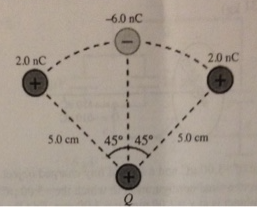# Problem: The point charge at the bottom of the figure is Q = +17 nC, and the curve is a circular arc. What is the magnitude of the force on the charge Q due to the other point charges shown? (k=1/4π ℇ0 = 8.99x109 N•m2/C2)

###### FREE Expert Solution

We use Coulomb's law in this problem given as:

$\overline{){\mathbf{F}}{\mathbf{=}}\frac{\mathbf{k}{\mathbf{q}}_{\mathbf{1}}{\mathbf{q}}_{\mathbf{2}}}{{\mathbf{r}}^{\mathbf{2}}}}$, where F is electric force, k is Coulomb's constant, and r is the separation between charges q1 and q2.

From Coulomb's law, the force due to charge 2.0 nC on Q is:

$\begin{array}{rcl}{\mathbf{F}}_{\mathbf{2}\mathbf{Q}}& \mathbf{=}& \frac{\mathbf{k}\mathbf{Q}\mathbf{\left(}\mathbf{2}\mathbf{.}\mathbf{0}\mathbf{×}{\mathbf{10}}^{\mathbf{-}\mathbf{9}}\mathbf{\right)}}{{\mathbf{r}}^{\mathbf{2}}}\mathbf{c}\mathbf{o}\mathbf{s}\mathbf{\theta }\end{array}$

96% (379 ratings)###### Problem Details

The point charge at the bottom of the figure is Q = +17 nC, and the curve is a circular arc. What is the magnitude of the force on the charge Q due to the other point charges shown? (k=1/4π ℇ0 = 8.99x109 N•m2/C2)